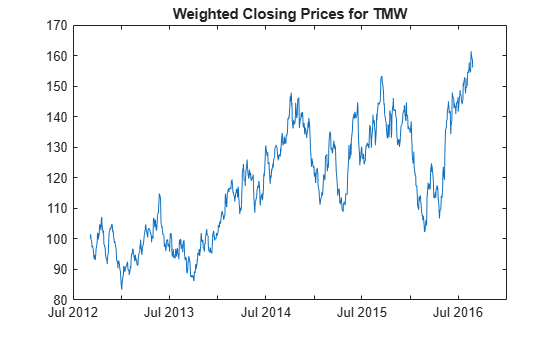# wclose

Weighted close

`wclose` has been partially removed and will no longer accept a `fints` object (`tsobj`) argument. Use a matrix, `timetable`, or `table` instead for financial time series.

Use `fts2timetable` to convert a `fints` object to a `timetable` object.

## Syntax

``WeightedClose = wclose(Data)``

## Description

example

````WeightedClose = wclose(Data)` calculates the weighted closing prices from the series of high, low, and closing prices. The weighted closing price is the average of twice the closing price plus the high and low prices.```

## Examples

collapse all

Load the file `SimulatedStock.mat`, which provides a timetable (`TMW`) for financial data for TMW stock.

```load SimulatedStock.mat WeightedClose = wclose(TMW); plot(WeightedClose.Time,WeightedClose.WeightedClose) title('Weighted Closing Prices for TMW')```## Input Arguments

collapse all

Data for high, low, and closing prices, specified as a matrix, table, or timetable. For matrix input, `Data` is an `M`-by-`3` matrix of high, low, and closing prices stored in the corresponding columns. Timetables and tables with `M` rows must contain variables named `'High'`, `'Low'`, and `'Close'` (case insensitive).

Data Types: `double` | `table` | `timetable`

## Output Arguments

collapse all

Weighted closing price series, returned with the same number of rows (`M`) and the same type (matrix, table, or timetable) as the input `Data`.

 Achelis, S. B. Technical Analysis from A to Z. Second Edition. McGraw-Hill, 1995, pp. 312–313.